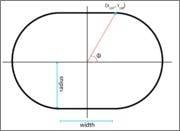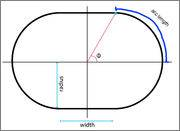# Arc length of a stadium billiard

carlosbgois
I've been trying to figure out the most straightforward way of doing this for a while, and would like to get some advice on new approaches, as the one I was using didn't work out at all. So here it is:

The stadium billiard is defined as two semicircles joined by two tangent lines, as shown in the figure attached. Given the angle of a point in the boundary, my goal is to determine the arc length of the boundary, from theta=0 to the collision angle. To do that, I have the parameters of the length of the tangent lines, and the radius of the semicircles (figure.) What approach would fit best here?

Thank you all for your time.Gold Member
2021 Award
I'm not following what it is that you want to figure out. Can you add to your figure the "arc length" you are talking about?

carlosbgois
Sorry about that. Here is an image showing what is the arc length I'm referring to:Staff Emeritus
Homework Helper
Sorry about that. Here is an image showing what is the arc length I'm referring to:Since the circle is tangent to the parallel lines, then the arc length from the start point to the tangent point, as indicated on your diagram is (π/2) * radius of the circle.

Gold Member
2021 Award
Since the circle is tangent to the parallel lines, then the arc length from the start point to the tangent point, as indicated on your diagram is (π/2) * radius of the circle.
But that's not true for the general case, only for when the angle is such that the point is at the exact meeting of the semicircle and the tangent. I'm reading this as his calling "arc" the amount you specified plus some amount of the tangent line.

I haven't worked on it yet but it appears to be trivial.

carlosbgois
Yes I'm looking for a general solution: given θ, what is the arc length?

Gold Member
2021 Award
Yes I'm looking for a general solution: given θ, what is the arc length?
Well, you know what the circle-arc part of it is. Can you use trivial trig to get the rest?

Staff Emeritus
Homework Helper
But that's not true for the general case, only for when the angle is such that the point is at the exact meeting of the semicircle and the tangent. I'm reading this as his calling "arc" the amount you specified plus some amount of the tangent line.

I haven't worked on it yet but it appears to be trivial.

Since the parallel lines are tangent to the circular arcs (as specified by the OP), and the parallel lines are exactly 2*radius apart, then the arc length as shown in the figure will be (π/2)*radius. The angle θ shown on the diagram is not referenced from the center of the circular arcs, so it does not have a direct relationship with the arc length.

For a student, it doesn't look completely trivial to me. Using the angle ##\phi## labeled in the picture and calling ##W = \frac 1 2\text{width}## where width is as labeled, and the radius of the circle ##a##, I get the arc length as$$s(\phi) = a(\phi + \arcsin\left(\frac W a\sin\phi\right)),~~0\le \phi \le \arctan\frac a W$$for just the first quadrant arc, which is the hard part.